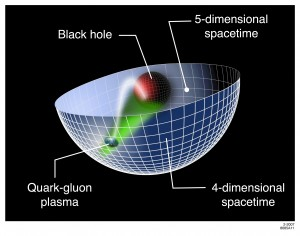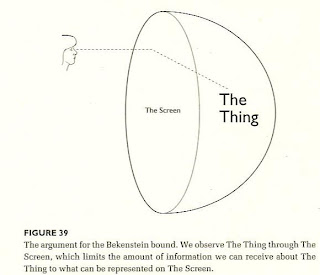## Wednesday, June 15, 2011

### A Conformal Field Theory Approach?

Using the anti–de Sitter/conformal field theory correspondence to relate fermionic quantum critical fields to a gravitational problem, we computed the spectral functions of fermions in the field theory. By increasing the fermion density away from the relativistic quantum critical point, a state emerges with all the features of the Fermi liquid. See:String Theory, Quantum Phase Transitions, and the Emergent Fermi LiquidSpacetime in String Theory Dr. Gary Horowitz, UCSB
.

Conformal Field Theory

A conformal field theory is a quantum field theory (or statistical mechanics model at the critical point) that is invariant under the conformal group. Conformal field theory is most often studied in two dimensions where there is a large group of local conformal transformations coming from holomorphic functions.

If your not sure what I mean,  have a look at what is happening on the surface of the sphere, as a means from which  a 2D description,  is describing the black hole in a 5d space. Have you seen this image before?String theorists describe the physics of black holes in five-dimensional spacetime. They found that these five-dimensional objects provide a good approximation of the quark-gluon plasma in one fewer dimension, a relationship similar to the one between a three-dimensional object and its two-dimensional shadow. Image: SLAC National Accelerator Laboratory
Recreating the conditions present just after the Big Bang has given experimentalists a glimpse into how the universe formed. Now, scientists have begun to see striking similarities between the properties of the early universe and a theory that aims to unite gravity with quantum mechanics, a long-standing goal for physicists.
“Combining calculations from experiments and theories could help us capture some universal characteristic of nature,” said MIT theoretical physicist Krishna Rajagopal, who discussed these possibilities at the recent Quark Matter conference in Annecy, France.

One millionth of a second after the Big Bang, the universe was a hot, dense sea of freely roaming particles called quarks and gluons. As the universe rapidly cooled, the particles joined together to form protons and neutrons, and the unique state of matter known as quark-gluon plasma disappeared.See: String theory may hold answers about quark-gluon plasma
***
Bekenstein Bound

TWO UNIVERSES of different dimension and obeying disparate physical laws are rendered completely equivalent by the holographic principle. Theorists have demonstrated this principle mathematically for a specific type of five-dimensional spacetime ("anti–de Sitter") and its four-dimensional boundary. In effect, the 5-D universe is recorded like a hologram on the 4-D surface at its periphery. Superstring theory rules in the 5-D spacetime, but a so-called conformal field theory of point particles operates on the 4-D hologram. A black hole in the 5-D spacetime is equivalent to hot radiation on the hologram--for example, the hole and the radiation have the same entropy even though the physical origin of the entropy is completely different for each case. Although these two descriptions of the universe seem utterly unalike, no experiment could distinguish between them, even in principle. by Jacob D. Bekenstein
***Consider any physical system, made of anything at all- let us call it, The Thing. We require only that The Thing can be enclosed within a finite boundary, which we shall call the Screen(Figure39). We would like to know as much as possible about The Thing. But we cannot touch it directly-we are restrictied to making measurements of it on The Screen. We may send any kind of radiation we like through The Screen, and record what ever changes result The Screen. The Bekenstein bound says that there is a general limit to how many yes/no questions we can answer about The Thing by making observations through The Screen that surrounds it. The number must be less then one quarter the area of The Screen, in Planck units. What if we ask more questions? The principle tells us that either of two things must happen. Either the area of the screen will increase, as a result of doing an experiment that ask questions beyond the limit; or the experiments we do that go beyond the limit will erase or invalidate, the answers to some of the previous questions. At no time can we know more about The thing than the limit, imposed by the area of the Screen.

Page 171 and 172 0f, Three Roads to Quantum Gravity by Lee Smolin

***Juan Maldacena:
The strings move in a five-dimensional curved space-time with a boundary. The boundary corresponds to the usual four dimensions, and the fifth dimension describes the motion away from this boundary into the interior of the curved space-time. In this five-dimensional space-time, there is a strong gravitational field pulling objects away from the boundary, and as a result time flows more slowly far away from the boundary than close to it. This also implies that an object that has a fixed proper size in the interior can appear to have a different size when viewed from the boundary (Fig. 1). Strings existing in the five-dimensional space-time can even look point-like when they are close to the boundary. Polchinski and Strassler1 show that when an energetic four-dimensional particle (such as an electron) is scattered from these strings (describing protons), the main contribution comes from a string that is close to the boundary and it is therefore seen as a point-like object. So a string-like interpretation of a proton is not at odds with the observation that there are point-like objects inside it.

***

Holography encodes the information in a region of space onto a surface one dimension lower. It sees to be the property of gravity, as is shown by the fact that the area of th event horizon measures the number of internal states of a blackhole, holography would be a one-to-one correspondance between states in our four dimensional world and states in higher dimensions. From a positivist viewpoint, one cannot distinquish which discription is more fundamental.

Pg 198, The Universe in Nutshell, by Stephen Hawking

***

In 1919, Kaluza sent Albert Einstein a preprint --- later published in 1921 --- that considered the extension of general relativity to five dimensions. He assumed that the 5-dimensional field equations were simply the higher-dimensional version of the vacuum Einstein equation, and that all the metric components were independent of the fifth coordinate. The later assumption came to be known as the cylinder condition. This resulted in something remarkable: the fifteen higher-dimension field equations naturally broke into a set of ten formulae governing a tensor field representing gravity, four describing a vector field representing electromagnetism, and one wave equation for a scalar field. Furthermore, if the scalar field was constant, the vector field equations were just Maxwell's equations in vacuo, and the tensor field equations were the 4-dimensional Einstein field equations sourced by an EM field. In one fell swoop, Kaluza had written down a single covariant field theory in five dimensions that yielded the four dimensional theories of general relativity and electromagnetism. Naturally, Einstein was very interested in this preprint .(sorry link now dead)